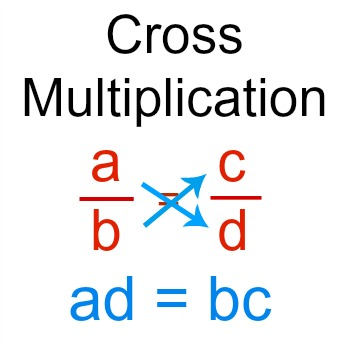# Which value makes the ratios equal: 4 to 6 and x/48?

Apr 4, 2018

$x = 32$

#### Explanation:

$\frac{4}{6} = \frac{x}{48} \rightarrow$ Set the ratios equal to one another

$\frac{4}{6} = \frac{2}{3} \rightarrow$ Simplify the first fraction

$\frac{2}{3} = \frac{x}{48} \rightarrow$ Cross multiply

$2 \cdot 48 = 3 \cdot x$

$96 = 3 x$

$x = 32$

Apr 4, 2018

$x = 32$

Here's how I did it:

#### Explanation:

To solve this, we set up a proportion:
$\frac{4}{6} = \frac{x}{48}$

Now, we use this method called cross multiplication:(study.com)

As you can see from this image, we multiply $4$ with $48$ and $6$ with $x$:
$4 \cdot 48 = 6 x$

$192 = 6 x$

And finally divide both sides by $6$:
$x = 32$

Hope this helps!